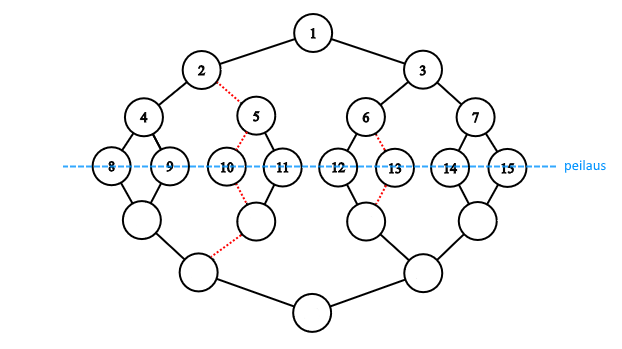CSES - Datatähti Open 2019 - Binary tree
• Time limit: 1.00 s
• Memory limit: 512 MB
A binary tree has $n$ levels and the tree is perfect, i.e., each node has two children (except for the leaves). The nodes of the tree are numbered so that the root is $1$ and the left and right children of a node $k$ are $2k$ and $2k+1$. In addition, the tree has $m$ forbidden edges that we may not traverse.

We construct a graph based on the tree by mirroring it in the leaves. How many paths are there such that the path starts and ends in the root of the original tree, it contains at least one edge and does not traverse any edge more than once?

For example, the following graph has $12$ such paths:Input

The first input line has two integers $n$ and $m$: the height of the tree and the number of forbidden edges.

After this, there are $m$ lines that contain integers $a_1, a_2, \dots, a_m$. An integer $a_i$ indicates that we may not use the edge between the nodes $a_i$ and $\left \lfloor{\frac{a_i}{2}}\right \rfloor$. Each such edge is given only once.

Output

Print one integer: the number of distinct paths modulo $10^9+7$.

Example

Input:
4 3 10 5 13

Output:
12

The image corresponds to the example. The forbidden edges are red.

• $2 \leq n \leq 6$
• $2 \leq n \leq 60$
• $m = 0$
• $2 \leq n \leq 60$
• $0 \leq m \leq 10^5$